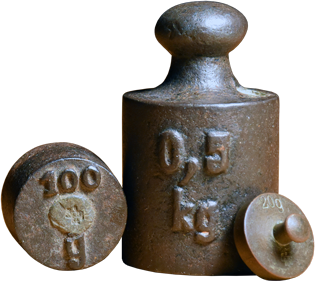# Definition of Metric SystemThe metric system is a system of weights and measures that has been adopted by many countries of the world.

Australia started to adopt the metric system in 1970.

The standard metric units are:

• length: the metre
• mass: the kilogram
• time: the second

For example,

• There are $1,000$ millimetres in a metre.
• There are $1,000$ kilograms in a tonne.
• There are $10,000$ square metres in a hectare.
• $1$ centimetre is $\dfrac{1}{100}$ metres.
• $1$ cubic centimetre is equal to $1$ millilitre.

### Description

The aim of this dictionary is to provide definitions to common mathematical terms. Students learn a new math skill every week at school, sometimes just before they start a new skill, if they want to look at what a specific term means, this is where this dictionary will become handy and a go-to guide for a student.

### Audience

Year 1 to Year 12 students

### Learning Objectives

Learn common math terms starting with letter M

Author: Subject Coach
You must be logged in as Student to ask a Question.